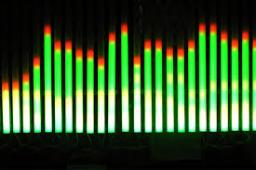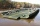# Equation

Solve equation and check the result:

1.4x - 3/2 + x - 9,8 = x + 0,4/3 - 7 + 1,6/6

Result

x =  3.357

#### Solution:

1.4x - 3/2 + x - 9.8 = x + 0.4/3 - 7 + 1.6/6

8.4x = 28.2

x = 4714 ≈ 3.357143

Calculated by our simple equation calculator.Our examples were largely sent or created by pupils and students themselves. Therefore, we would be pleased if you could send us any errors you found, spelling mistakes, or rephasing the example. Thank you!

Leave us a comment of this math problem and its solution (i.e. if it is still somewhat unclear...):Be the first to comment!#### Following knowledge from mathematics are needed to solve this word math problem:

Need help calculate sum, simplify or multiply fractions? Try our fraction calculator. Do you have a linear equation or system of equations and looking for its solution? Or do you have quadratic equation?

## Next similar math problems:

1. EqnSolve equation with fractions: 2x/3-50=40+x/4
2. Fraction + eqSolve following simple equation with fractions: -5/6(8+5b) = 75 + 5/3b
3. Simple equation 9Solve the following equation: -8y+5=-9y+9
4. Equation 29Solve next equation: 2 ( 2x + 3 ) = 8 ( 1 - x) -5 ( x -2 )
5. Unknown numberI think the number. I'll reduce it to its one-third. The result is then increased by one-third, and I get the number 12.
6. Grandmother and grandfatherGrandmother baked cakes. Grandfather ate half, then quarter of the rest ate Peter and Paul ate half of rest. For parents left 6 cakes. How many cakes maked the grandmother?
7. Unknown number 6Determine the unknown number, which is by 1.5 greater than its fourth.
8. Find unknownFind unknown numerator: 4/8 + _/8 = 1
9. New bridgeThanks to the new bridge, the road between A and B has been cut to one third and is now 10km long. How much did the road between A and B measure before?
10. PearsThere were pears in the basket, I took two-fifths of them, and left six in the basket. How many pears did I take?
11. Equation 20In given equation: 8/9-4/5=2/9+x, find x
12. ZdeněkZdeněk picked up 15 l of water from a 100-liter full-water barrel. Write a fraction of what part of Zdeněk's water he picked.
13. Write 2Write 791 thousandths as fraction in expanded form.
14. In fractionsAn ant climbs 2/5 of the pole on the first hour and climbs 1/4 of the pole on the next hour. What part of the pole does the ant climb in two hours?
15. Fraction and a decimalWrite as a fraction and a decimal. One and two plus three and five hundredths
16. Lengths of the poolMiguel swam 6 lengths of the pool. Mat swam 3 times as far as Miguel. Lionel swam 1/3 as far as Miguel. How many lengths did mat swim?
17. Fraction to decimalWrite the fraction 3/22 as a decimal.